Ex 5.3

Chapter 5 Class 10 Arithmetic Progressions (Term 2)
Serial order wise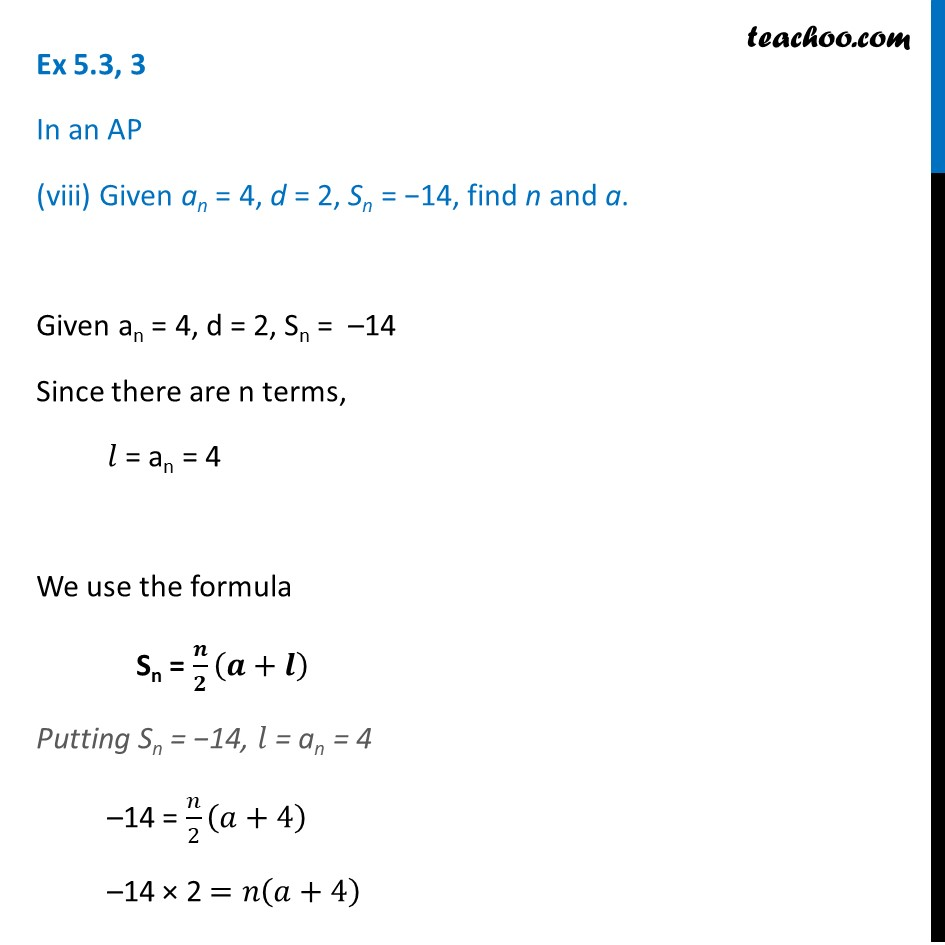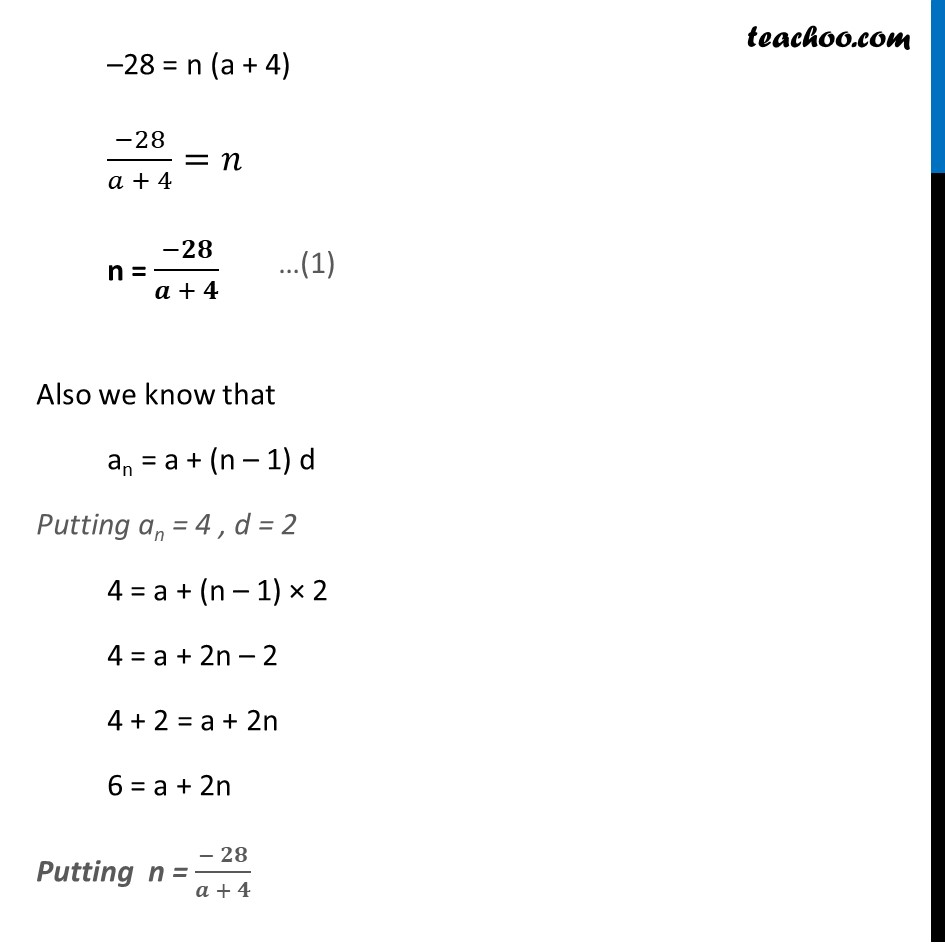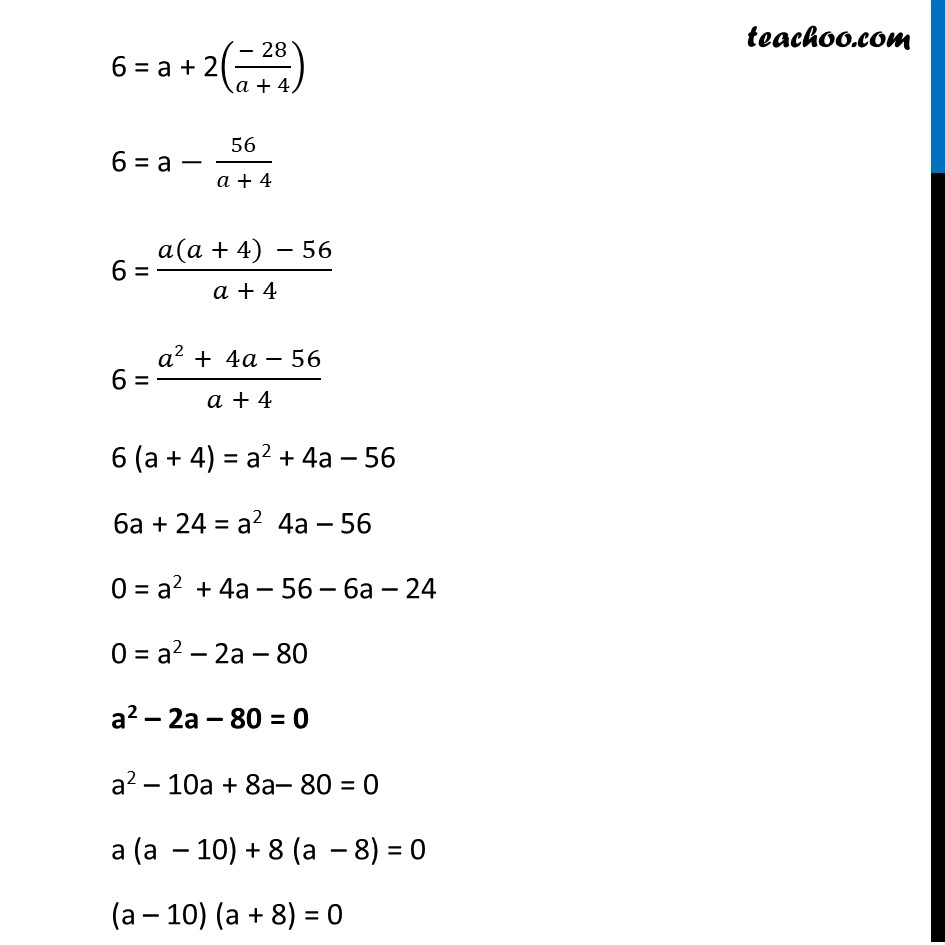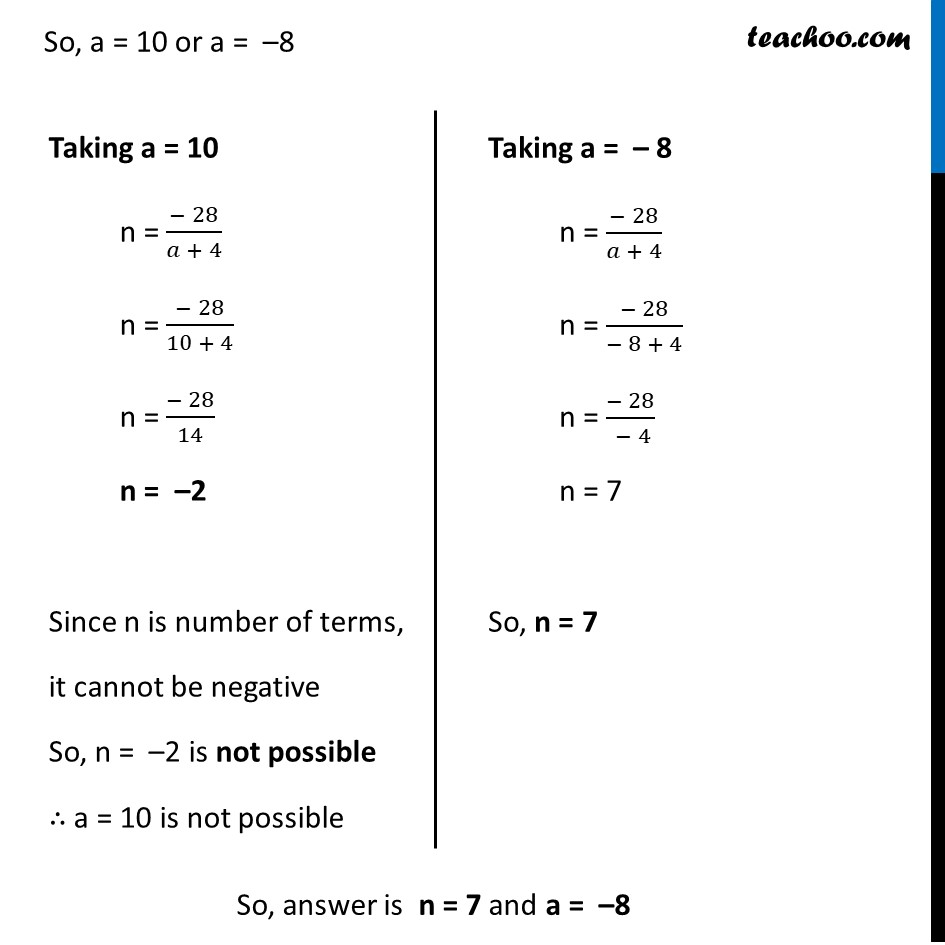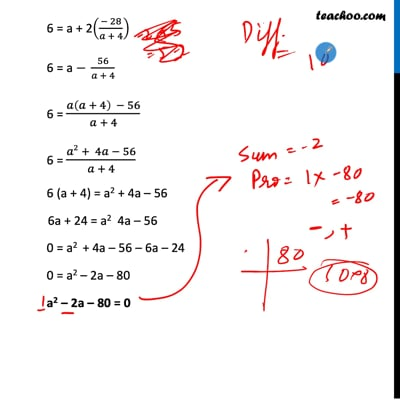This video is only available for Teachoo black users

### Transcript

Ex 5.3, 3 In an AP (viii) Given an = 4, d = 2, Sn = −14, find n and a. Given an = 4, d = 2, Sn = –14 Since there are n terms, 𝑙 = an = 4 We use the formula Sn = 𝒏/𝟐 (𝒂+𝒍) Putting Sn = −14, 𝑙 = an = 4 –14 = 𝑛/2 (𝑎+4) –14 × 2 =𝑛(𝑎+4) –28 = n (a + 4) (−28)/(𝑎 + 4)=𝑛 n = (−𝟐𝟖)/(𝒂 + 𝟒) Also we know that an = a + (n – 1) d Putting an = 4 , d = 2 4 = a + (n – 1) × 2 4 = a + 2n – 2 4 + 2 = a + 2n 6 = a + 2n Putting n = (− 𝟐𝟖)/(𝒂 + 𝟒) 6 = a + 2((− 28)/(𝑎 + 4)) 6 = a − 56/(𝑎 + 4) 6 = (𝑎(𝑎 + 4) − 56)/(𝑎 + 4) 6 = (𝑎2 + 4𝑎 − 56)/(𝑎 + 4) 6 (a + 4) = a2 + 4a – 56 6a + 24 = a2 4a – 56 0 = a2 + 4a – 56 – 6a – 24 0 = a2 – 2a – 80 a2 – 2a – 80 = 0 a2 – 10a + 8a– 80 = 0 a (a – 10) + 8 (a – 8) = 0 (a – 10) (a + 8) = 0 So, a = 10 or a = –8 Taking a = 10 n = (− 28)/(𝑎 + 4) n = (− 28)/(10 + 4) n = (− 28)/14 n = –2 Since n is number of terms, it cannot be negative So, n = –2 is not possible ∴ a = 10 is not possible Taking a = – 8 n = (− 28)/(𝑎 + 4) n = (− 28)/(− 8 + 4) n = (− 28)/( − 4) n = 7 So, n = 7